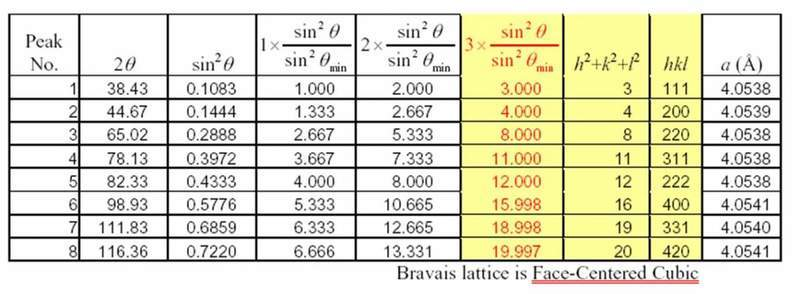# Determine crystal lattice structure from powder XRD

## Homework Statement

Using the powder XRD data below, show that the substance has a face centred cubic structure. (xray lamda = 0.154056 nm)

Peak No.------2(theta)
1 -------------38.06
2 -------------44.24
3 -------------64.34
4 -------------68.77
5 -------------73.07

## Homework Equations

$$2dsin\theta = n\lambda$$

$$d = \frac{a}{\sqrt{N}}$$

$$\Delta sin\theta = \left(\frac{\lambda}{4a^{2}}\right)N_{2} - N_{1}$$

$$N= h^{2}+k^{2}+l^{2}$$

## The Attempt at a Solution

I've worked out sin theta for each sin theta squared and delta sin theta:

Peak------2(theta)------sin theta----sin squared theta---delta sin squared theta
1-----------38.06-------0.32606-------0.10632------------
2-----------44.24-------0.37655-------0.14179------------0.03547
3-----------64.34-------0.53243-------0.28349------------0.1417
4-----------68.77-------0.56475-------0.31894------------0.03545
5-----------73.07-------0.59531-------0.35440------------0.03549

The only example we've covered is with a primitive cubic structure which I almost knew what I was doing(!) and the only advice that the lecturer gave was to "look for the highest common factor of values in the list delta sin squared theta to find $$\frac{\lambda}{4a^{2}}$$

I obviously noted that the difference between peak 2 and 3 was the same value as Peak 2 but what I'm meant to do with that information I'm not so sure about!!?

I know that a fcc structure only has N values of 3,4,8,11 etc but really could do with some advice as where to go from here!!?

Any help at all would be appreciated!!! I've spent ages searching for answers and the people that i've spolen to at uni have no clue either so i'd love to be able to pass on the knowledge!!!

## Homework Statement

Using the powder XRD data below, show that the substance has a face centred cubic structure. (xray lamda = 0.154056 nm)

Peak No.------2(theta)
1 -------------38.06
2 -------------44.24
3 -------------64.34
4 -------------68.77
5 -------------73.07

## Homework Equations

$$2dsin\theta = n\lambda$$

$$d = \frac{a}{\sqrt{N}}$$

$$\Delta sin\theta = \left(\frac{\lambda}{4a^{2}}\right)N_{2} - N_{1}$$

$$N= h^{2}+k^{2}+l^{2}$$

## The Attempt at a Solution

I've worked out sin theta for each sin theta squared and delta sin theta:

Peak------2(theta)------sin theta----sin squared theta---delta sin squared theta
1-----------38.06-------0.32606-------0.10632------------
2-----------44.24-------0.37655-------0.14179------------0.03547
3-----------64.34-------0.53243-------0.28349------------0.1417
4-----------68.77-------0.56475-------0.31894------------0.03545
5-----------73.07-------0.59531-------0.35440------------0.03549

The only example we've covered is with a primitive cubic structure which I almost knew what I was doing(!) and the only advice that the lecturer gave was to "look for the highest common factor of values in the list delta sin squared theta to find $$\frac{\lambda}{4a^{2}}$$

I obviously noted that the difference between peak 2 and 3 was the same value as Peak 2 but what I'm meant to do with that information I'm not so sure about!!?

I know that a fcc structure only has N values of 3,4,8,11 etc but really could do with some advice as where to go from here!!?
hai
i want to know what is the formula for finding a,b,c using 'd' and 2theta values. also if the structure is known how to calculate miller indices for the corresponding peaks

For Cubic crystal finding hkl (miller indices) is easy note that peak in xrd is a importent factor
(1) Identify the peaks.
(2) Determine sin2$\theta$
(3) Calculate the ratio sin2$\theta$/ sin2$\theta$min and multiply by the appropriate integers.
(4) Select the result from (3) that yields h2 + k2 + l2 as an integer.
(5) Compare results with the sequences of h2 + k2 + l2 values to identify the Bravais lattice.
(6) Calculate lattice parameters.

Identify the peaks and their proper 2 values. Eight peaks for this pattern.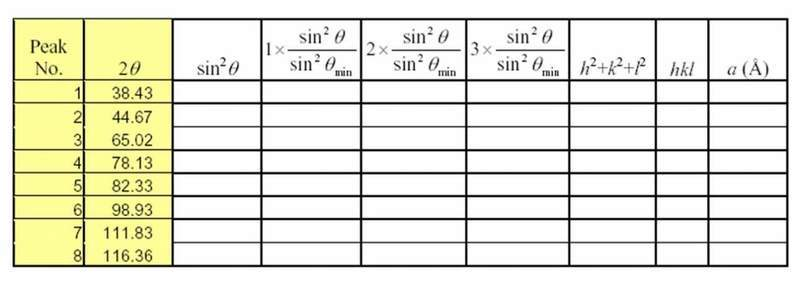Determine sin2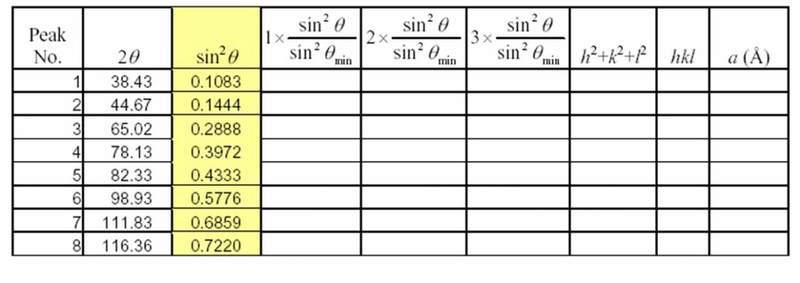Calculate the ratio sin2/ sin2min and multiply by the appropriate integers.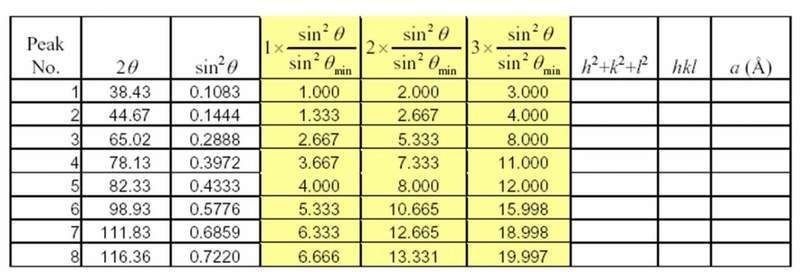Select the result from (3) that yields h2 + k2 + l2 as a series of integers.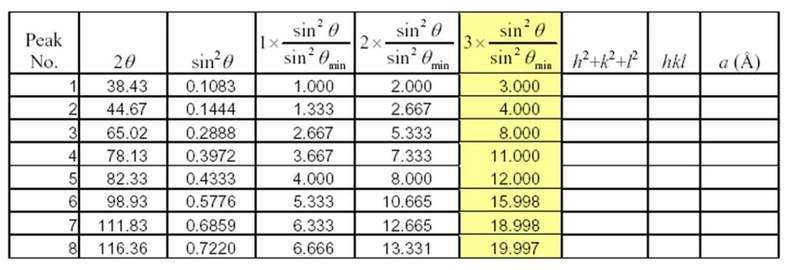Compare results with the sequences of h2 + k2 + l2 values to identify the Bravais lattice.